# Uplink Carrier Aggregation Waveform Generation, Demodulation, and Analysis

This example shows how multiple uplink carriers can be generated, aggregated, and demodulated using LTE Toolbox™. Error Vector Magnitude (EVM) and in-band emissions are measured for the demodulated carrier as per TS 36.101 Annex F [ 1 ].

### Introduction

This example models an LTE uplink waveform with Carrier Aggregation (CA). The number of component carriers (CC) and their respective bandwidths can be specified as a parameter. An intra-band contiguous CA case is considered as per TS 36.101 V15.4.0.

To generate an aggregated uplink waveform a user equipment (UE) is configured for each component carrier. Carrier aggregation parameters are calculated and used to generate a modulated waveform for each CC configuration. All the component carrier modulated waveforms are resampled to a common sampling rate so that they can be combined to create an aggregated waveform. The aggregated waveform is demodulated and filtered to extract the component carrier of interest. Then EVM and in-band emissions are measured for that CC and finally a CRC check is made by performing PUSCH decoding.

### Number of Component Carriers and Bandwidths

A vector NULRB specifies the number of resource blocks (RBs) for each component carrier. The length of this vector corresponds to the number of CCs. The elements of NULRB must be in the set {6, 15, 25, 50, 75, 100} RBs. TS 36.101 Table 5.6A.1-1 [ 1 ] lists the valid combinations of bandwidths for carrier aggregation.

% Configure the set of NULRB values to describe the carriers to be % aggregated NULRB = [50 75 100]; % Establish the number of component carriers numCC = numel(NULRB); CCBWs = zeros(1,numCC); if numCC<2 error('Please specify more than one CC bandwidth value.'); end 

### UE Configuration for each Component Carrier

A configuration structure is generated for each CC using lteRMCUL. To show the in-band emissions, partial RB allocation is being considered for each CC. The configuration structures for all CCs are stored in a cell array.

% Specify the number of subframes to generate numSubframes = 10; % Configure CCs frc = cell(1,numCC); for i = 1:numCC switch NULRB(i) case 6 frc{i} = lteRMCUL('A3-1'); frc{i}.PUSCH.PRBSet = (0:2).'; case 15 frc{i} = lteRMCUL('A1-2'); frc{i}.PUSCH.PRBSet = (0:8).'; case 25 frc{i} = lteRMCUL('A1-3'); frc{i}.PUSCH.PRBSet = (0:15).'; case 50 frc{i} = lteRMCUL('A3-5'); frc{i}.PUSCH.PRBSet = (0:39).'; case 75 frc{i} = lteRMCUL('A3-6'); frc{i}.PUSCH.PRBSet = (0:59).'; case 100 frc{i} = lteRMCUL('A3-7'); frc{i}.PUSCH.PRBSet = (0:80).'; otherwise fprintf('Not a valid number of resource blocks: %d\n',... NULRB(i)); return end CCBWs(i) = hNRBToBandwidth(NULRB(i)); frc{i}.Bandwidth = CCBWs(i); frc{i}.TotSubframes = numSubframes; frc{i}.PUSCH.RVSeq = 0; end 

### Channel Estimator Configuration

The channel estimator at the receiver end is parameterized using the structure cec defined below.

cec = struct; % Channel estimation config structure cec.PilotAverage = 'UserDefined'; % Type of pilot symbol averaging cec.FreqWindow = 15; % Frequency window size cec.TimeWindow = 15; % Time window size cec.InterpType = 'Cubic'; % 2D interpolation type cec.InterpWindow = 'Centered'; % Interpolation window type cec.InterpWinSize = 1; % Interpolation window size cec.Reference = 'Antennas'; % Specifies point of reference for % channel estimation. 

### Carrier Aggregation Parameters Calculation

To perform carrier aggregation the frequency parameters described in TS 36.101, Sections 5.6 and 5.7 [ 1 ] are calculated. These parameters are summarized in the following figure.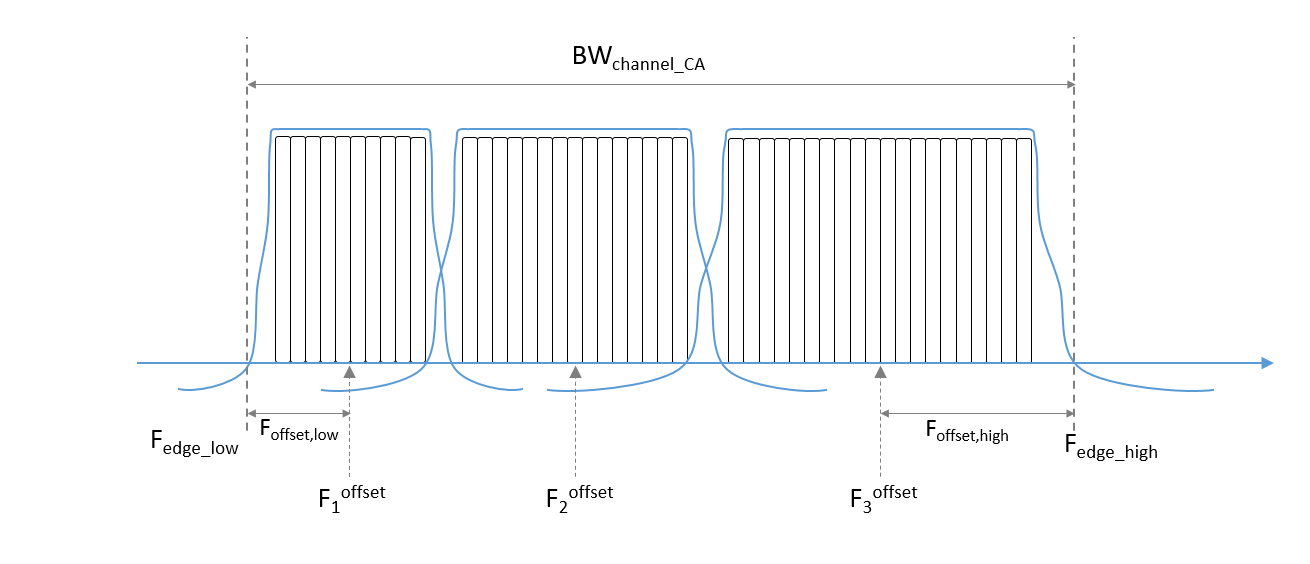This results in three variables:

• F_c is a vector containing the center frequency of each CC

• ccSpacing contains the spacing between CCs in MHz

• BW_channel_CA is the aggregated channel bandwidth of all CCs

In the code below we first calculate the value for all the CCs assuming the lower one is centered at baseband (0 Hz). Once the BW_channel_CA is calculated all the values are shifted so that the center of the aggregated bandwidth is located at baseband (0 Hz).

% Define Delta_f1 parameter in MHz for nominal guard band and frequency % offset calculations. In the uplink Delta_f1 is zero % (TS 36.101, Table 5.6A-1) Delta_f1 = 0; % in MHz maxBW = max(CCBWs); % Initialize center frequency and CC spacing vectors F_c = zeros(1,numCC); % Center frequencies ccSpacing = zeros(1,numCC-1); % CC spacing % Calculate nominal guard band, TS 36.101 5.6A-1 nominalGuardBand = 0.05*maxBW-0.5*Delta_f1; % Initially assume lower carrier frequency is at baseband F_c(1) = 0; % Calculate CC spacing and center frequencies for k = 2:numCC ccSpacing(k-1) = hCarrierAggregationChannelSpacing( ... frc{k-1}.Bandwidth, frc{k}.Bandwidth); F_c(k) = F_c(k-1) + ccSpacing(k-1); end % Calculate lower and higher frequency offsets, TS 36.101 5.6A F_offset_low = (0.18*NULRB(1)+Delta_f1)/2 + nominalGuardBand; F_offset_high = (0.18*NULRB(end)+Delta_f1)/2 + nominalGuardBand; % Calculate lower and higher frequency edges, TS 36.101 5.6A F_edge_low = F_c(1) - F_offset_low; F_edge_high = F_c(end) + F_offset_high; % Calculate aggregated channel bandwidth, TS 36.101 5.6A BW_channel_CA = F_edge_high - F_edge_low; fprintf('BW_channel_CA: %0.4f MHz\n',BW_channel_CA); % Calculate shift to center baseband shiftToCenter = -1*(BW_channel_CA/2 + F_edge_low); % Shift the center frequencies so that the aggregated bandwidth is centered % at baseband F_c = F_c + shiftToCenter; F_edge_low = F_c(1) - F_offset_low; F_edge_high = F_c(end) + F_offset_high; % Display frequency band edges fprintf('F_edge_low: %0.4f MHz\n',F_edge_low); fprintf('F_edge_high: %0.4f MHz\n',F_edge_high); fprintf('F_offset_low: %0.4f MHz\n',F_offset_low); fprintf('F_offset_high: %0.4f MHz\n',F_offset_high); % Display carrier frequencies fprintf('\n'); for i = 1:numCC fprintf('Component Carrier %d:\n',i); fprintf('Fc: %0.4f MHz\n', F_c(i)); end 
BW_channel_CA: 44.6000 MHz F_edge_low: -22.3000 MHz F_edge_high: 22.3000 MHz F_offset_low: 5.5000 MHz F_offset_high: 10.0000 MHz Component Carrier 1: Fc: -16.8000 MHz Component Carrier 2: Fc: -4.8000 MHz Component Carrier 3: Fc: 12.3000 MHz 

### Required Oversampling Rate Calculation

The required oversampling factors for each component carrier OSRs are calculated for a common sampling rate for the aggregated signal.

% Considering the bandwidth utilization as 85% bwfraction = 0.85; % Calculate sampling rates of the component carriers CCSR = zeros(1,numCC); for i = 1:numCC info = lteSCFDMAInfo(frc{i}); CCSR(i) = info.SamplingRate; end % Calculate overall sampling rate for the aggregated signal % Calculate the oversampling ratio (for the largest BW CC) to make sure the % signal occupies 85% (bwfraction) of the aggregated channel bandwidth OSR = (BW_channel_CA/bwfraction)/(max(CCSR)/1e6); % To simplify the resampling operation, choose an oversampling ratio as the % smallest power of 2 greater than or equal to OSR OSR = 2^ceil(log2(OSR)); SR = OSR*max(CCSR); fprintf('\nOutput sample rate: %0.4f Ms/s\n\n',SR/1e6); % Calculate individual oversampling factors for the component carriers OSRs = SR./CCSR; 
Output sample rate: 61.4400 Ms/s 

### Waveform Generation and Carrier Aggregation

lteRMCULTool is used to generate the waveform for each CC. These are resampled to a common sampling rate, frequency modulated to the appropriate center frequency, and finally added together to form the aggregated signal.

% Generate component carriers tx = cell(1,numCC); for i = 1:numCC tx{i} = lteRMCULTool(frc{i},randi([0 1],1000,1)); tx{i} = resample(tx{i},OSRs(i),1)/OSRs(i); tx{i} = hCarrierAggregationModulate(tx{i},SR,F_c(i)*1e6); end % Superpose the component carriers waveform = tx{1}; for i = 2:numCC waveform = waveform + tx{i}; end 

### Plot Carrier Aggregation Waveform Spectrum

The power spectrum of the carrier aggregated signal is displayed using hCarrierAggregationPlotSpectrum.m. In the spectrum the individual carrier bandwidths are visible. Note that the center of the aggregated bandwidth is located at baseband (0 Hz) as the signal is not modulated to RF in this example.

specPlot = hCarrierAggregationPlotSpectrum(waveform,SR,... 'Power Spectrum of Carrier Aggregation Waveform',... {'Carrier aggregated signal'});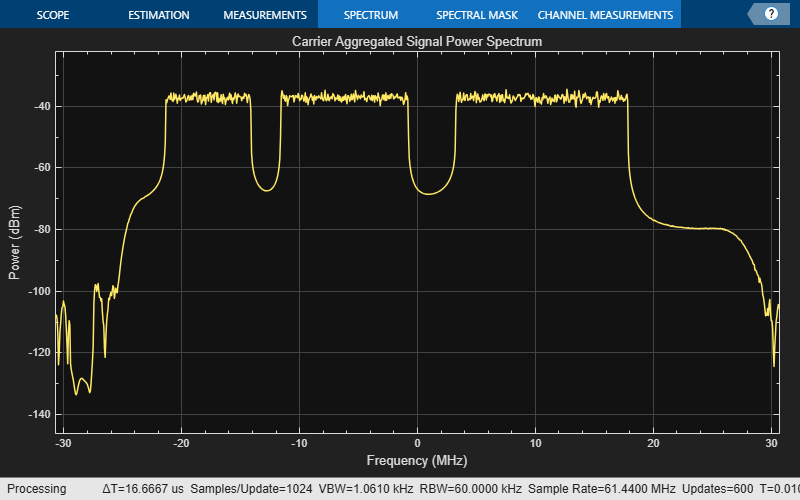### Demodulation and Filtering of CC of Interest

This section shows how to demodulate, filter, and downsample one of the CC of interest. The steps followed are:

• Demodulate the CC of interest and bring it to baseband (0 Hz).

• Filter out neighboring CCs and downsample. A suitable filter is designed to remove the unwanted neighboring CCs in the downsampling process. The filter design will have an impact on the quality and EVM of the recovered signal. Filters may need to be tweaked for different values of bandwidth and CC to demodulate.

We start by selecting the CC to demodulate and designing the appropriate downsampling filter with the calculated passband and stopband frequencies.

% Select CC to demodulate CCofInterest = 1; if CCofInterest > numCC || CCofInterest <= 0 error('Cannot demodulate CC number %d, there are %d CCs\n',... CCofInterest, numCC) ; end % Define downsampling filter order filterOrder = 301; % Precalculate passband and stopband values for all CC firPassbandVec = (0.18*NULRB+Delta_f1)/2 / (SR/1e6/2); firStopbandVec = hCarrierAggregationStopband(ccSpacing,NULRB,SR); % Define passband and stopband for carrier of interest firPassband = firPassbandVec(CCofInterest); firStopband = firStopbandVec(CCofInterest); % Extract and decode CC of interest fprintf(1,'Extracting CC number %d: \n', CCofInterest); % Pad signal with zeros to take into account filter transient response % length waveform = [waveform; zeros(filterOrder+1,size(waveform,2))]; % Demodulate carrier of interest demodulatedCC = ... hCarrierAggregationModulate(waveform,SR,-F_c(CCofInterest)*1e6); % Downsampling is done in two stages if the filter is too narrow. This % eases the filter design requirements. If this is the case an initial % downsampling factor of 4 is applied. You may want to consider a different % filter design in this initial stage if the quality of the resulting % signal is not acceptable. if (firStopband < 0.1) % Down-sample by 4 SRC = 4; demodulatedCC = resample(demodulatedCC,1,SRC); % Update pass and stopband to take first downsampling into account firPassband = firPassband * SRC; firStopband = firStopband * SRC; else % No pre-filter SRC = 1; end % Design lowpass filter to filter out CC of interest frEdges = [0 firPassband firStopband 1]; firFilter = dsp.FIRFilter; firFilter.Numerator = firpm(filterOrder,frEdges,[1 1 0 0]); 
Extracting CC number 1: 

The response of the designed filter is displayed.

fvtool(firFilter,'Analysis','freq');The demodulated waveform is then filtered to extract the CC of interest. The spectrum of the demodulated waveform before and after the filtering is plotted.

% Filter the signal to extract the component of interest rxWaveform = firFilter(demodulatedCC); % Plot the demodulated and filtered signal spectra filteredSpecPlot = ... hCarrierAggregationPlotSpectrum([demodulatedCC, rxWaveform],SR,... 'Power Spectrum of Demodulated and Filtered Waveform',... {'Carrier aggregated signal' 'Filtered signal'});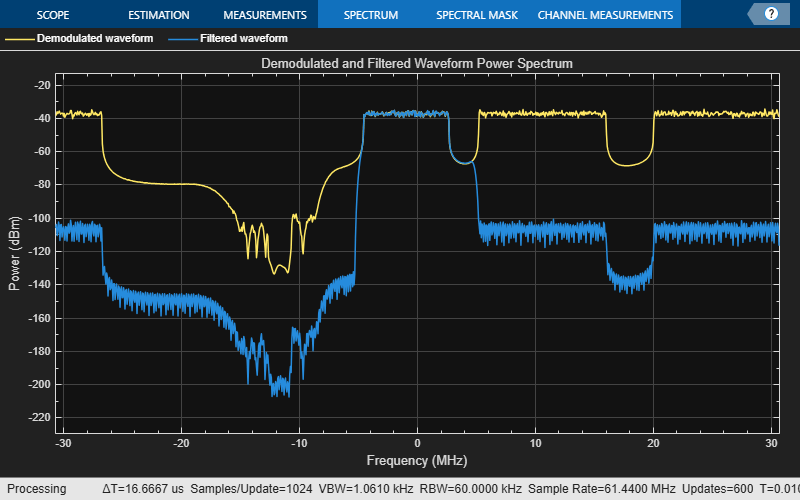At this point the filtered waveform can be downsampled to its baseband rate.

rxWaveform = downsample(rxWaveform,OSRs(CCofInterest)/SRC); 

### Synchronization

Synchronization is applied to the resulting signal before EVM and in-band emissions measurement.

% Get the parameters for CC of interest FRC = frc{CCofInterest}; PUSCH = FRC.PUSCH; % Synchronize received waveform offset = lteULFrameOffset(FRC,PUSCH,rxWaveform); rxWaveform = rxWaveform(1+offset:end, :); 

### EVM, In-Band Emissions Measurements, and PUSCH Decoding

The code below provides PUSCH EVM, PUSCH DRS EVM, and in-band emissions calculation using hPUSCHEVM.

The EVM is the difference between the reconstructed ideal symbols and measured received symbols over one slot measurement interval in the time domain. The process of reconstructing the ideal symbols includes:

• Single-carrier frequency division multiple access (SC-FDMA) demodulation to obtain the received resource grid

• Channel estimation

• PUSCH equalization

• Symbol demodulation

• Decoding followed by reencoding of the received bits, rescrambling and remodulation

The average EVM is measured at two locations in time (low and high, which are denoted asand), where the low and high locations correspond to the alignment of the FFT window within the start and end of the cyclic prefix. LTE Toolbox requires the low and high locations to be specified as a fraction of the cyclic prefix length.

The in-band emissions are a measure of the interference falling into the non-allocated RBs. In-band emissions are calculated for eachand slot number, whereis the starting frequency offset between the allocated RB and the measured non-allocated RB (e.g.,for the first adjacent RB outside of the allocated bandwidth).

From the above measurements the following plots are also produced:

• EVM versus OFDM symbol

• EVM versus subcarrier

• EVM versus resource block

• EVM versus OFDM symbol and subcarrier (i.e. the EVM resource grid)

• Uplink in-band emissions for non-allocated RBs

Note that the EVM measurements displayed at the command window are only calculated across allocated PUSCH resource blocks, in accordance with the LTE standard. The EVM plots are shown across all resource blocks (allocated or unallocated), allowing the in-band emissions to be viewed. In unallocated resource blocks, the EVM is calculated assuming that the received resource elements have an expected value of zero.

The PUSCH of the recovered signal is then decoded and the resulting CRC is checked for errors.

% Configure channel estimation structure for EVM measurement cecEVM = cec; cecEVM.PilotAverage = 'TestEVM'; [evmpusch, evmdrs, emissions, plots] = hPUSCHEVM(frc{CCofInterest},cecEVM,rxWaveform); % Plot the absolute in-band emissions if (~isempty(emissions.DeltaRB)) hPUSCHEVMEmissionsPlot(emissions); end % Perform SC-FDMA demodulation rxGrid = lteSCFDMADemodulate(FRC,rxWaveform); % Get the number of received subframes and OFDM symbols per subframe dims = lteSCFDMAInfo(FRC); samplesPerSubframe = dims.SamplingRate/1000; nRxSubframes = floor(size(rxWaveform, 1)/samplesPerSubframe); FRC.TotSubframes = 1; resGridSize = lteResourceGridSize(FRC); L = resGridSize(2); disp('Decode transmitted subframes and check CRC.'); for n=0:nRxSubframes-1 % Extract subframe rxSubframe = rxGrid(:,(1:L)+(n*L),:); % Get the transport block size for current subframe FRC.NSubframe = n; trBlkSize = PUSCH.TrBlkSizes(n+1); % Perform channel estimation [estChannelGrid, noiseEst] = lteULChannelEstimate( ... FRC, PUSCH, cec, rxSubframe); % Generate PUSCH indices and extract symbols from received and % channel estimate grids in preparation for PUSCH decoding ind = ltePUSCHIndices(FRC, PUSCH); [rxSym,hestSym] = lteExtractResources(ind, rxSubframe, estChannelGrid); % Update PUSCH to carry complete information of the UL-SCH coding PUSCH = lteULSCHInfo(FRC, PUSCH, trBlkSize, 'chsconcat'); % Perform equalization, transform deprecoding, layer demapping, % demodulation, and descrambling on the received data using the channel % estimate [rxEncodedBits, puschsymbols] = ltePUSCHDecode(FRC, PUSCH, ... rxSym, hestSym, noiseEst); % Decode Uplink Shared Channel (UL-SCH) decState = []; [decbits,crc] = ... lteULSCHDecode(FRC, PUSCH, trBlkSize ,rxEncodedBits, decState); if crc disp(['Subframe ' num2str(n) ': CRC failed']); else disp(['Subframe ' num2str(n) ': CRC passed']); end end 
Low edge PUSCH EVM, slot 0: 0.165% Low edge PUSCH EVM, slot 1: 0.244% Low edge DRS EVM, slot 0: 0.210% Low edge DRS EVM, slot 1: 0.199% High edge PUSCH EVM, slot 0: 0.264% High edge PUSCH EVM, slot 1: 0.253% High edge DRS EVM, slot 0: 0.208% High edge DRS EVM, slot 1: 0.242% Low edge PUSCH EVM, slot 2: 0.213% Low edge PUSCH EVM, slot 3: 0.175% Low edge DRS EVM, slot 2: 0.186% Low edge DRS EVM, slot 3: 0.307% High edge PUSCH EVM, slot 2: 0.220% High edge PUSCH EVM, slot 3: 0.258% High edge DRS EVM, slot 2: 0.264% High edge DRS EVM, slot 3: 0.367% Low edge PUSCH EVM, slot 4: 0.225% Low edge PUSCH EVM, slot 5: 0.175% Low edge DRS EVM, slot 4: 0.180% Low edge DRS EVM, slot 5: 0.236% High edge PUSCH EVM, slot 4: 0.260% High edge PUSCH EVM, slot 5: 0.243% High edge DRS EVM, slot 4: 0.234% High edge DRS EVM, slot 5: 0.261% Low edge PUSCH EVM, slot 6: 0.228% Low edge PUSCH EVM, slot 7: 0.194% Low edge DRS EVM, slot 6: 0.294% Low edge DRS EVM, slot 7: 0.149% High edge PUSCH EVM, slot 6: 0.306% High edge PUSCH EVM, slot 7: 0.243% High edge DRS EVM, slot 6: 0.290% High edge DRS EVM, slot 7: 0.216% Low edge PUSCH EVM, slot 8: 0.205% Low edge PUSCH EVM, slot 9: 0.203% Low edge DRS EVM, slot 8: 0.269% Low edge DRS EVM, slot 9: 0.164% High edge PUSCH EVM, slot 8: 0.244% High edge PUSCH EVM, slot 9: 0.268% High edge DRS EVM, slot 8: 0.342% High edge DRS EVM, slot 9: 0.201% Low edge PUSCH EVM, slot 10: 0.184% Low edge PUSCH EVM, slot 11: 0.249% Low edge DRS EVM, slot 10: 0.211% Low edge DRS EVM, slot 11: 0.203% High edge PUSCH EVM, slot 10: 0.246% High edge PUSCH EVM, slot 11: 0.327% High edge DRS EVM, slot 10: 0.206% High edge DRS EVM, slot 11: 0.410% Low edge PUSCH EVM, slot 12: 0.221% Low edge PUSCH EVM, slot 13: 0.273% Low edge DRS EVM, slot 12: 0.170% Low edge DRS EVM, slot 13: 0.255% High edge PUSCH EVM, slot 12: 0.322% High edge PUSCH EVM, slot 13: 0.285% High edge DRS EVM, slot 12: 0.297% High edge DRS EVM, slot 13: 0.362% Low edge PUSCH EVM, slot 14: 0.215% Low edge PUSCH EVM, slot 15: 0.214% Low edge DRS EVM, slot 14: 0.274% Low edge DRS EVM, slot 15: 0.183% High edge PUSCH EVM, slot 14: 0.232% High edge PUSCH EVM, slot 15: 0.253% High edge DRS EVM, slot 14: 0.405% High edge DRS EVM, slot 15: 0.251% Low edge PUSCH EVM, slot 16: 0.224% Low edge PUSCH EVM, slot 17: 0.240% Low edge DRS EVM, slot 16: 0.278% Low edge DRS EVM, slot 17: 0.260% High edge PUSCH EVM, slot 16: 0.248% High edge PUSCH EVM, slot 17: 0.310% High edge DRS EVM, slot 16: 0.197% High edge DRS EVM, slot 17: 0.409% Low edge PUSCH EVM, slot 18: 0.290% Low edge PUSCH EVM, slot 19: 0.214% Low edge DRS EVM, slot 18: 0.279% Low edge DRS EVM, slot 19: 0.190% High edge PUSCH EVM, slot 18: 0.304% High edge PUSCH EVM, slot 19: 0.261% High edge DRS EVM, slot 18: 0.335% High edge DRS EVM, slot 19: 0.264% Averaged low edge PUSCH EVM, frame 0: 0.220% Averaged high edge PUSCH EVM, frame 0: 0.269% Averaged PUSCH EVM frame 0: 0.269% Averaged DRS EVM frame 0: 0.297% Averaged overall PUSCH EVM: 0.269% Averaged overall DRS EVM: 0.297% Decode transmitted subframes and check CRC. Subframe 0: CRC passed Subframe 1: CRC passed Subframe 2: CRC passed Subframe 3: CRC passed Subframe 4: CRC passed Subframe 5: CRC passed Subframe 6: CRC passed Subframe 7: CRC passed Subframe 8: CRC passed Subframe 9: CRC passed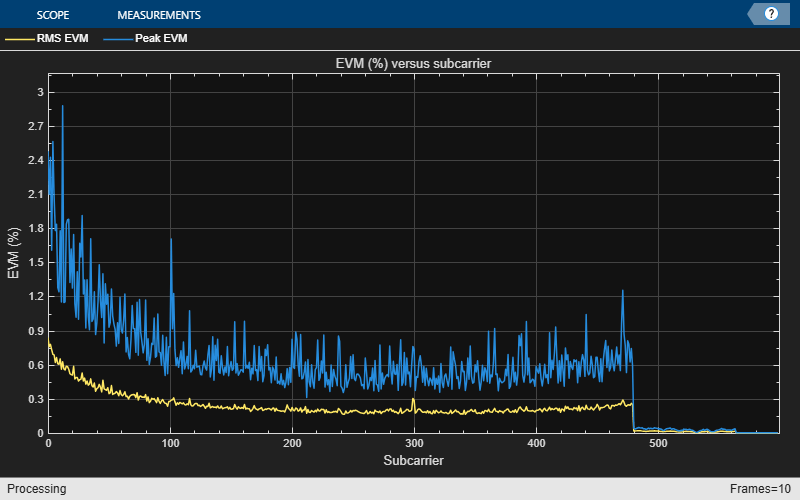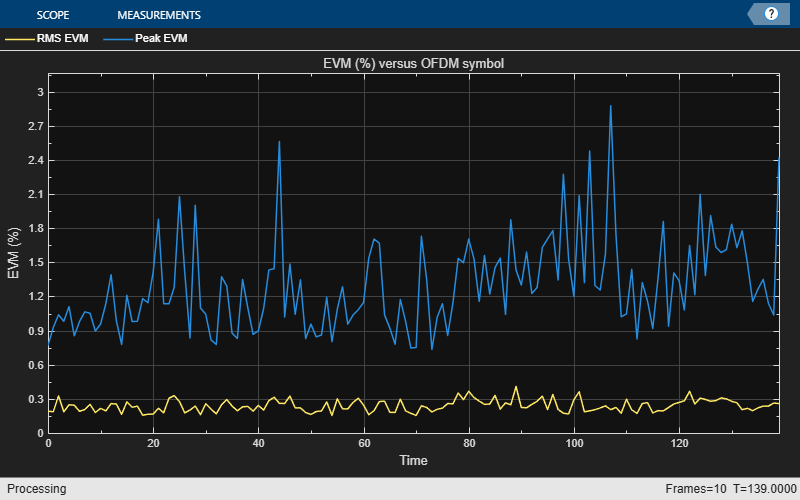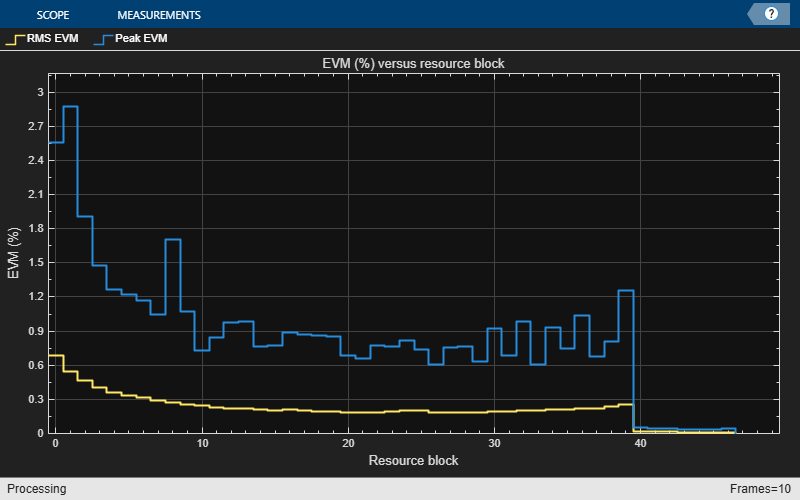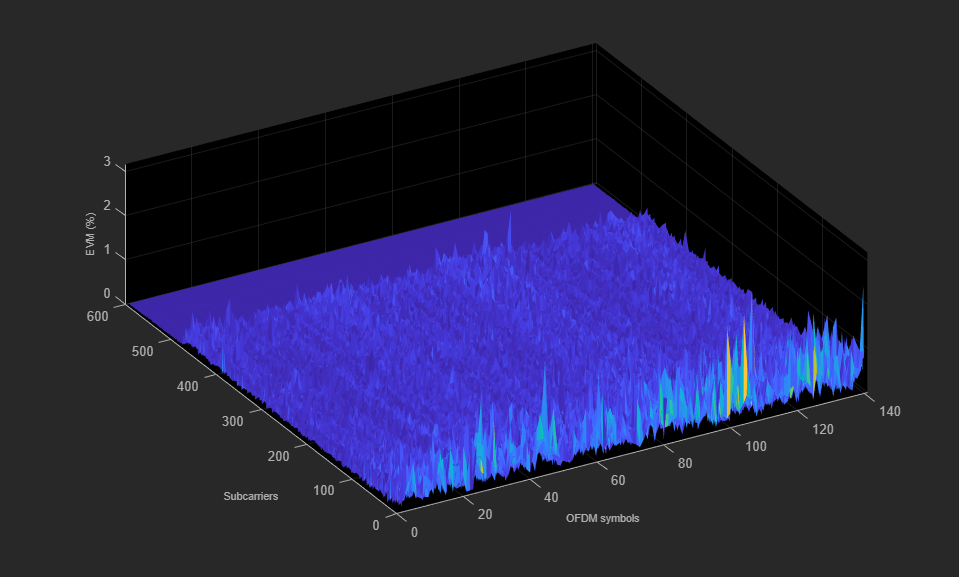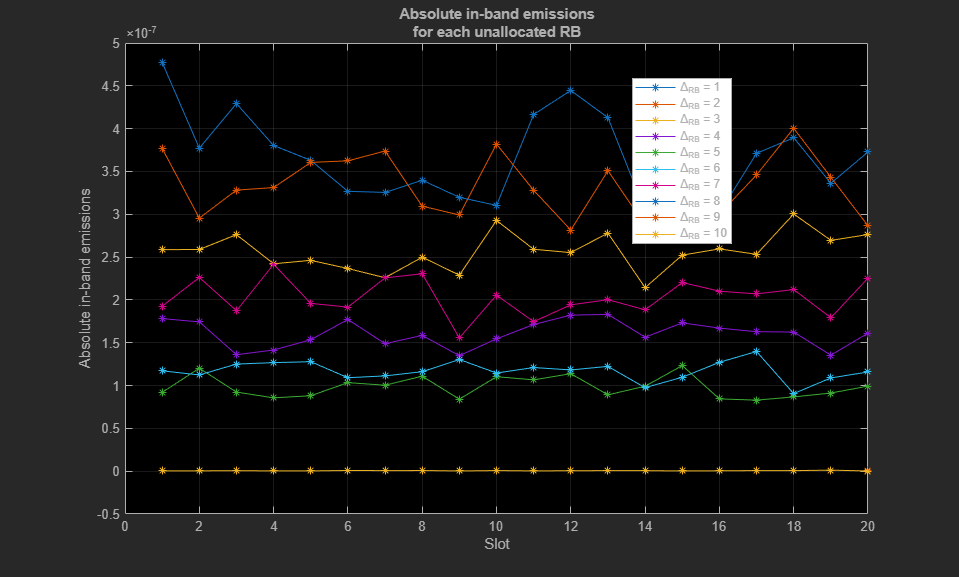### Appendix

This example uses the following helper functions:

### Selected Bibliography

1. 3GPP TS 36.101 "User Equipment (UE) radio transmission and reception"